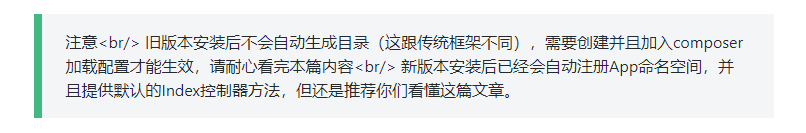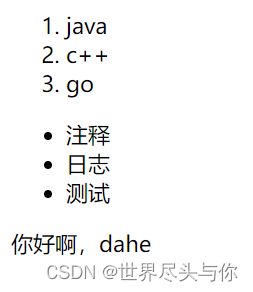# thinkPHP 模板中的语法知识 详细介绍(十二)

+关注继续查看

<script src='__PUBLIC__/Js/test.js'></script>
2.import（==默认是在Public文件夹下）
//导入Public文件夹下面的Js目录中的test.js文件，import标签可以省略type属性，默认就是js的
<import type='js' file='Js.test' />
<import type='css' file='Css.test' />

//可以更改默认文件夹 设置basepath属性
//就会在 根目录下找到 Other/Js/my.js,就不是默认的Public目录
<import type='js' file='Js.my' basepath='./Other'/>
//方法可以自动检测导入的文件类型

1、if
<if condition='$sex eq "男"'> 男人哭吧哭吧不是罪！ <else /> 做女人挺好的！ </if> <if condition='$age lt 18'>

<elseif  condition='$age eq 18'/> 奋斗吧少年！ <else /> 成年 </if> > gt < lt == eq <= elt >= egt != neq === heq !== nheq <switch name='myAge'> //变量不要加$  直接名字myAge 默认会加$<case value='1'>太小啦你</case> <case value='2'>你也太小</case> <case value='30'>你还差不多</case> <default/> 这里是默认值 </switch> 三、循环结构 1.for <table border='1' width='500'> /* start（必须）：循环变量开始值 end（必须）：循环变量结束值 name（可选）：循环变量名，默认值为i step（可选）：步进值，默认值为1,自加！ comparison（可选）：判断条件，默认为lt */ 就是我们php for中的i <for start='0' end='10' name='j' step='2'> <tr><td>{$j}</td><td>abc</td></tr>
</for>
//倒序
<for start='10' end='00' name='j' step='-2' comparison='gt'>
<tr><td>{$j}</td><td>abc</td></tr> </for> </table> 2.volist（数组遍历） <volist name='list' id='v'> {$v.username}<br/>
</volist>
//从下表=1的开始取2条
<volist name='list' id='v' offset='1' length='2'>
<{$v}><br> </volist> //遍历多维数组 <volist name='arrd' id='v'> <{$v.id}>--<{$v.username}><br> </volist> 3.foreach <foreach name='list' item='v' key='k'> {$k}-------{$v}<br/> </foreach> 四、特殊标签(手册也有详细介绍哦) 1、比较标签 eq或者 equal 等于 模板中：//等同于if else的使用 number传的变量 <eq name='number' value='10'> 如果传的name=10我就输出这里也！ <else/> 不等于10我就输出这里哦！ </eq> neq 或者notequal 不等于 gt 大于 egt 大于等于 lt 小于 elt 小于等于 heq 恒等于 nheq 不恒等于 2.范围标签(类似if else逻辑和书写方式) in <in name='number' value='9,10,11,12'> 在这些数字里面 <else/> 不在这些数字的范围内 </in> <notin name='number' value='9,10,11,12'> 在这些数字里面 <else/> 不在这些数字的范围内 </notin> between //1-10之间 <notbetween name='number' value='1,10'> {$number}在1-10之间
<else/>
{\$number}不在1到10之间
</between>

notbetween-->标签来判断变量不在某个范围内
<notbetween name="id"value="1,10">输出内容1</notbetween>

3.present

<present name='m'>m有赋值<else/>m没有赋值</present>
4.Empty
empty标签判断模板变量是否为空，
<empty name='number'>number为空赋值<else/>number有值</empty>
5.Defined

6.Define

7.Assing

1、*在模板中直接使用PHP代码
<php> echo "我是乐杨俊" </php>  //注意里面只能写php的代码，嵌入tp的标签是不能用的
2、建议更改左右定界符

'TMPL_L_DELIM'=>'<{', //修改左定界符
'TMPL_R_DELIM'=>'}>', //修改右定界符|
12月前
|
PHP
PHP解析Markdown库,parsedown扩展自定义语法

179 0|
12月前
|

【Gin】模板的高级用法

266 1|

93 0
|
JavaScript

315 0
thinkphp5常用模板标签使用方法
thinkphp5常用模板标签使用方法
225 0
|
PHP
thinkphp模板if里面使用函数示例
thinkphp模板if里面使用函数示例
139 0
|

1229 0